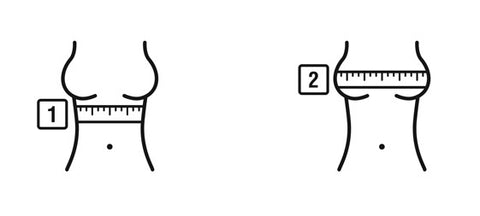Mini Cart

• No products in the cart.

WHAT IS MY BAND SIZE?Knowing your band size is essential to a good fit.

EZ-Fit method

What do the numbers mean?
The numbers - like 28, 30, 32, 34, 36, 38, are band sizes –
The band is the part of the bra that goes around our rib cage1. Measure under the Bust to determine band measurement

Measure around directly under bust & around the rib cage round to nearest whole number to determine band size

 If you measure your rib cage as 29 inches around your band size is 30 If you measure your rib cage as 27 inches around your band size is 28 If you measure your rib cage as 34 inches around your band size is 34 If you measure your rib cage as 30 inches around your band size is 30 If you measure your rib cage as 33 inches around your band size is 34 If you measure your rib cage as 35 inches around your band size is 36 If you measure your rib cage as 36 inches around your band size is 36 If you measure your rib cage as 37 inches around your band size is 38

*Band measurements always round up to nearest even number

Band measurement is compared to Full bust measurement to determine bra cup size.

Measure across the Bust to determine cup size - measure the fullest part of the bust holding tape parallel with the floor to determine cup
size

BUST measurement - BAND SIZE = CUP SIZE inches
1 inch = A cup
2 inch = B cup
3 inch = C cup
4 inch = D cup
5 inch = DD cup
What do the letters mean?
The letters - like A, B, C, D, DD,- stand for cup sizes.

SOME EXAMPLES

A full-bust measurement of 35 is 5 inches larger than a 30 band measurement.
35 - 30 = 5 inches
These 5 inches are equivalent to a “DD” cup size; and a final bra size of 30DD.
1 inch = A cup, 2 inch = B cup, 3 inch = C cup, 4 inch = D cup, 5 inch = DD cup, 6 inch = DDD cup

A full-bust measurement of 36 is 4 inches larger than a 32 band measurement
36 - 32 = 4 inches
These 4 inches are equivalent to a “D” cup size; and a final bra size of 32D.
1 inch = A cup, 2 inch = B cup, 3 inch = C cup, 4 inch = D cup, 5 inch = DD cup, 6 inch = DDD cup